1 篇文章 0 订阅

# 深拷贝与浅拷贝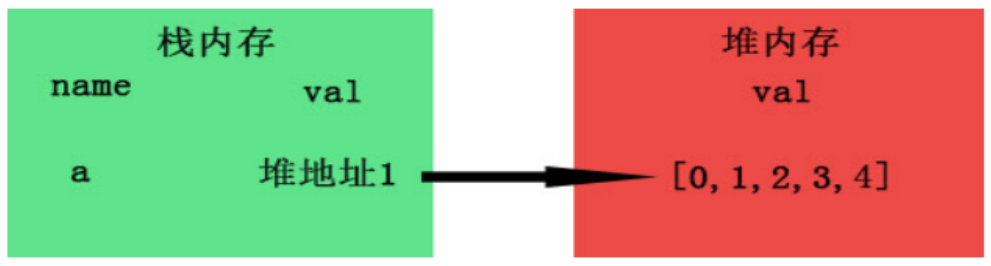b = a 进行拷贝时，复制的是 a 的引用地址，而并非堆里的值。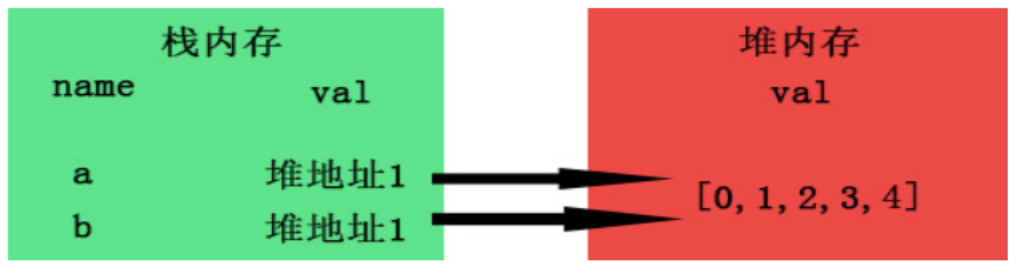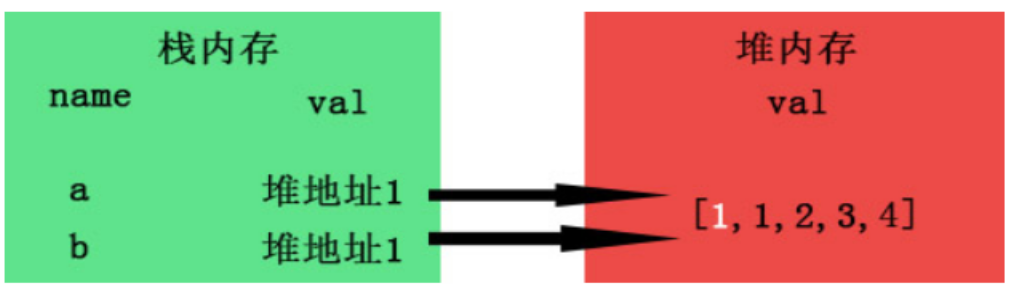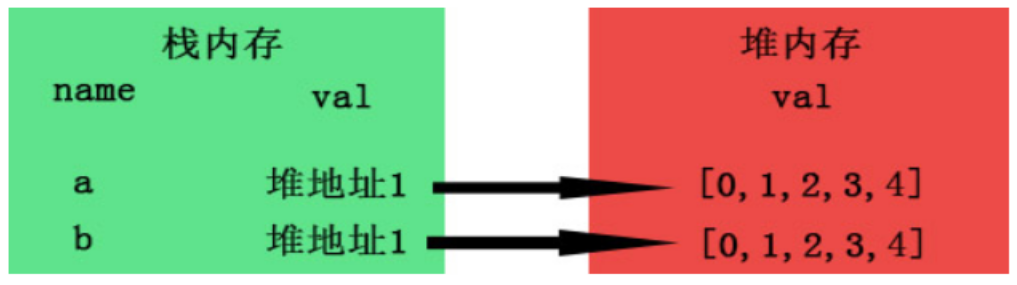## 实现深拷贝的方法

### 递归

function deepClone (obj) {
let objClone = Array.isArray(obj)?[]:{};
if(obj && typeof obj === 'object'){
for(key in obj){
if(obj.hasOwnProperty(key)){
//判断obj的子元素是否为对象，如果是，递归复制
if(obj[key] && typeof obj[key] === 'object'){
objClone[key] = deepClone(obj[key]);
}else{
//如果不是，简单复制
objClone[key] = obj[key];
}
}
}
}
return objClone;
}
let a = [1,2,{name: 'zhangsan',age: 24},4]
b = deepClone(a)
a = 2;
a.age = 26
console.log(a,b)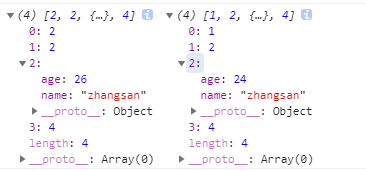let a = [1,2,3,4];
let b = a.slice();
a = 2;
console.log(a,b)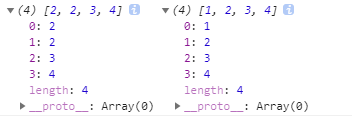let a = [1,2,[3,4],5];
let b = a;
a = 2;
a = 6;
console.log(a,b)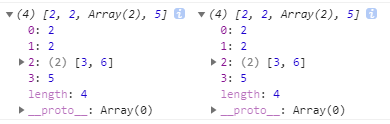### JSON对象的parse和stringify

function deepClone (obj) {
let _obj = JSON.stringify(obj);
let objClone = JSON.parse(_obj);
return objClone
}
let a = [1,2,[3,4],5];
let b = deepClone(a);
a = 2;
a = 6;
console.log(a,b)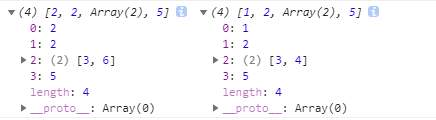function deepClone (obj) {
let _obj = JSON.stringify(obj);
let objClone = JSON.parse(_obj);
return objClone
}
let a = {
name: 'xxx',
eat: function () {
},
age: undefined,
};
let b = deepClone(a);
console.log(a)
console.log(b)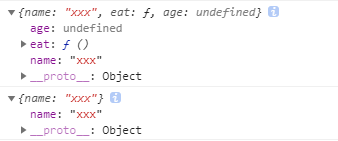function deepClone (obj) {
let _obj = JSON.stringify(obj);
let objClone = JSON.parse(_obj);
return objClone
}
let b = deepClone(a);
console.log(a)
console.log(b)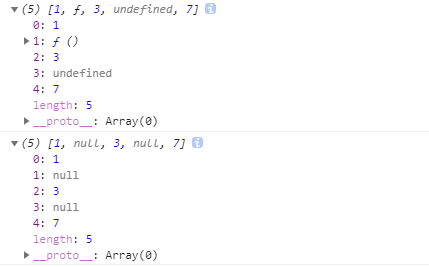### Jquery的extend方法

$.extend([deep],target.object1,object2)deepboolean 值，true 为深拷贝，false 为浅拷贝，target.object1 为目标对象，object2 为需要拷贝的对象。 let a = [0,1,[2,3],4]; let b =$.extend(true,[],a);
a = 1;
a = 5;
console.log(a,b);

let d = $.extend(true,[],c); console.log(c,d); let e = { name: 'xxx', eat: function () { alert('吃饭了') }, age: undefined, book: null, }; let f =$.extend(true,[],e);
console.log(e,f);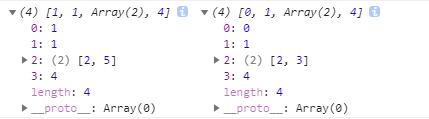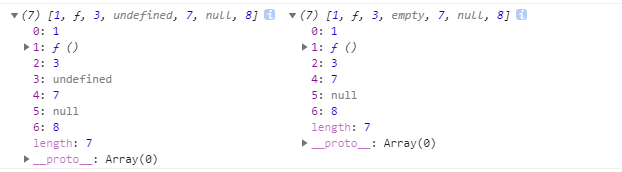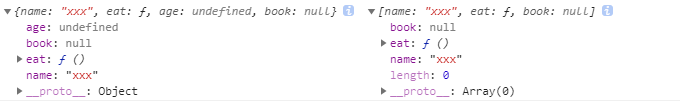09-05
08-31
10-14
10-21834
03-011895
09-01点击重新获取扫码支付1.余额是钱包充值的虚拟货币，按照1:1的比例进行支付金额的抵扣。
2.余额无法直接购买下载，可以购买VIP、C币套餐、付费专栏及课程。余额充值